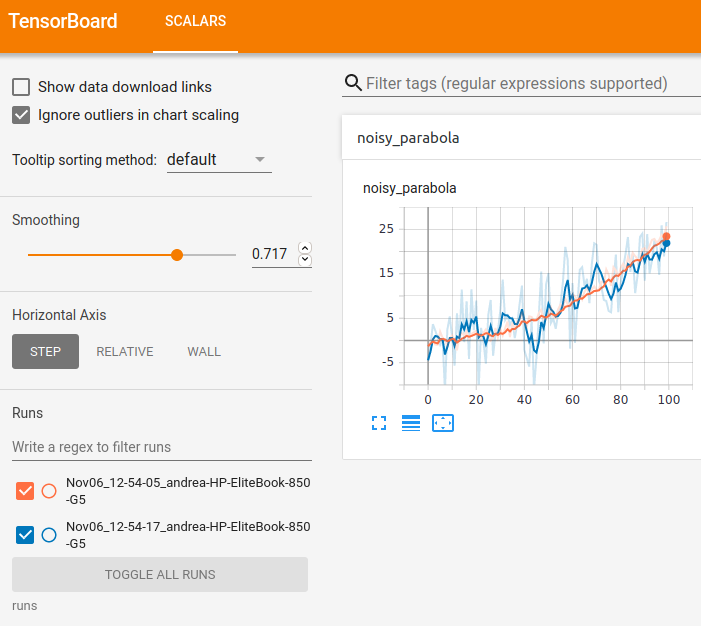Collection of snippets of `tensorboard` usage with `pytorch` using the tensorboardX library.

## Requirements

``````pip install torch
pip install tensorflow
pip install tensorboard
``````

## Run tensorboard

``````tensorboard --logdir runs
``````

Open up the webapp in your browser, usually at `http://localhost:6006`.

## Training script

``````import numpy as np
from torch.utils.tensorboard import SummaryWriter

## clean run folder
import shutil
import time
shutil.rmtree('runs', ignore_errors=True)

## run 1
writer = SummaryWriter(flush_secs=1)
for x in range(100):
noise = np.random.normal(scale=1)
y = (x/20)**2 + noise
time.sleep(0.1)
writer.close()

## run 2
writer = SummaryWriter(flush_secs=1)
for x in range(100):
noise = np.random.normal(scale=6)
y = (x/20)**2 + noise